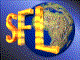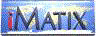| iMatix home page | << | < | > | >>SFLVersion 2.11

### tree_prev

```#include "sfltree.h"
void *tree_prev (void *tree)
```

#### Synopsis

Finds and returns the previous node in a tree.

#### Source Code - (sfltree.c)

```{
TREE
*current,
*child;

if ((!tree)
||  (tree == TREE_NULL))
return NULL;

current = tree;
if (current-> left != TREE_NULL)
return tree last (current-> left);
else
{
current = tree;
child   = TREE_NULL;
while ((current-> parent)
&&  (current-> left == child))
{
child = current;
current = current-> parent;
}
if (current-> left != child)
return current;
else
return NULL;
}
}
```

 | << | < | > | >>Copyright © 1996-2000 iMatix Corporation